# Overview

Let’s design a RAPTOR flowchart to compute the sum of squares for n integers. The RAPTOR flowchart enables us to create executable flowcharts.

## Mathematical Notation

Let’s first understand mathematical notation. The mathematical notation for the sum of squares up to n numbers is as follows:

or in simple notation

Mathematical verification formula:

sum of squares = n(n+1)(2n + 1)/6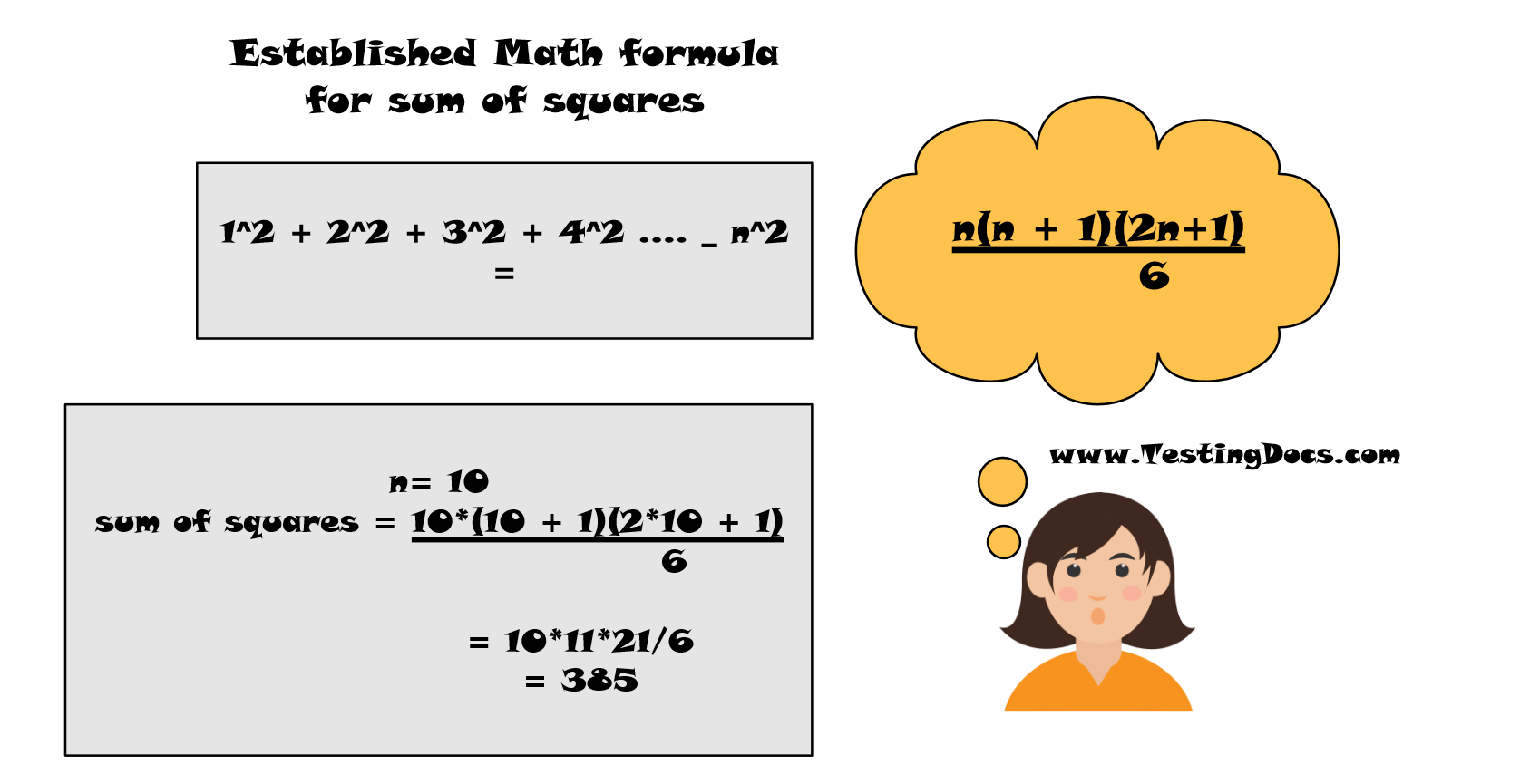## Steps to build

The general steps to build the RAPTOR flowchart are as follows:

• Launch RAPTOR Flowchart software. A blank flowchart with Start and End symbols would be provided.
• Save the flowchart with a .rap file extension name.
• Add an Assignment flowchart symbol for Assignment( left-click once on the symbol and then add it between Start and End.)
• Initialize the following variables

i <- 1
sum <- 0
series <- ” ”

• Add an Input symbol to the flowchart. Double click to add the prompt “Enter number” and use the variable name N.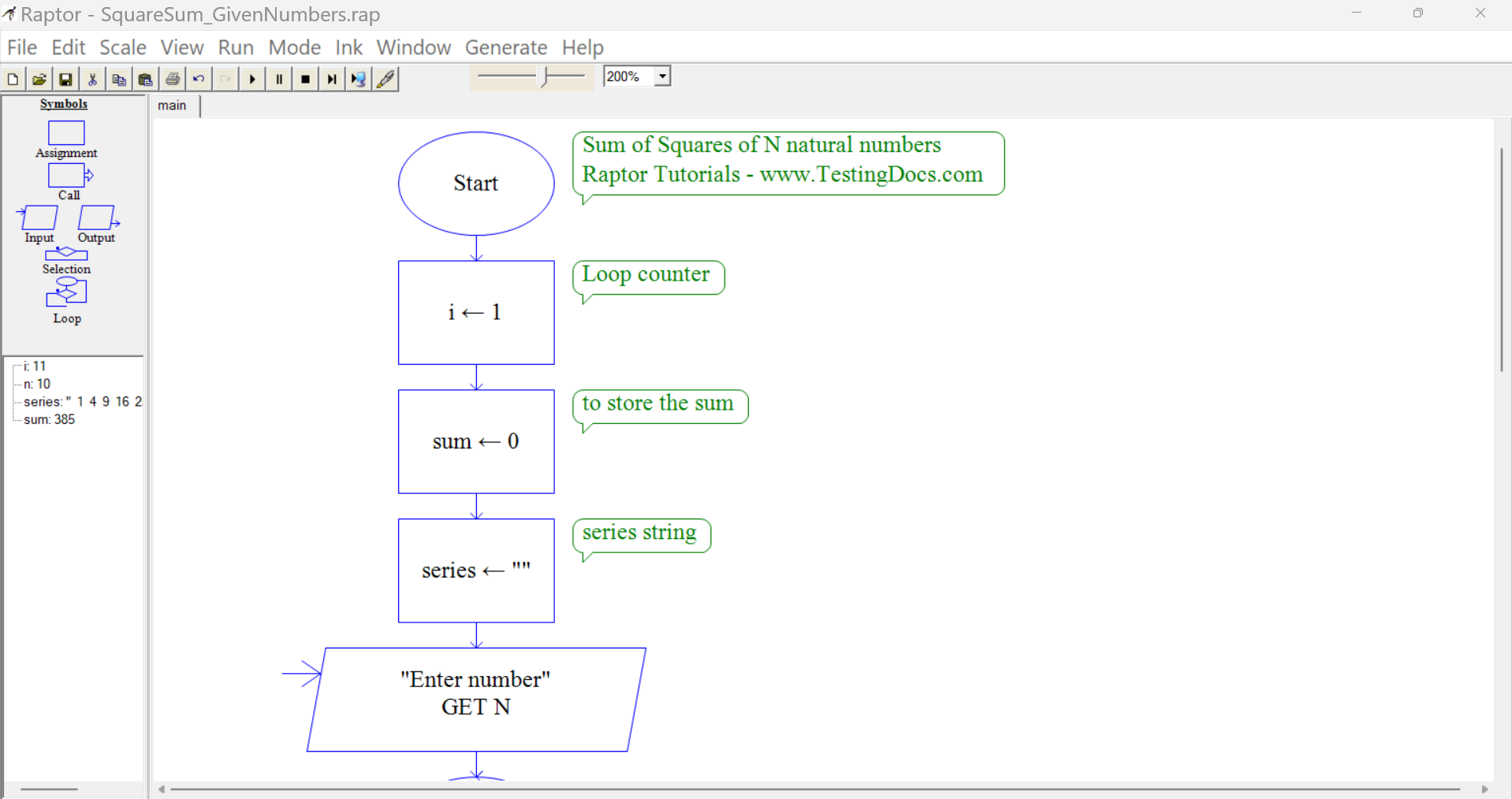## Loop Structure

i > N

• In the loop structure for NO decision, do the following things:
• Add Assignment symbols to the flowchart to the flowchart for sum, series and i

sum <- sum + ( i* i )
series <- series + ” ” + ( i*i )
i <- i + 1

• Add an Output symbol to the flowchart to output series

series <- series + ” ” + ( i*i ) statement is to print the series to the output console window.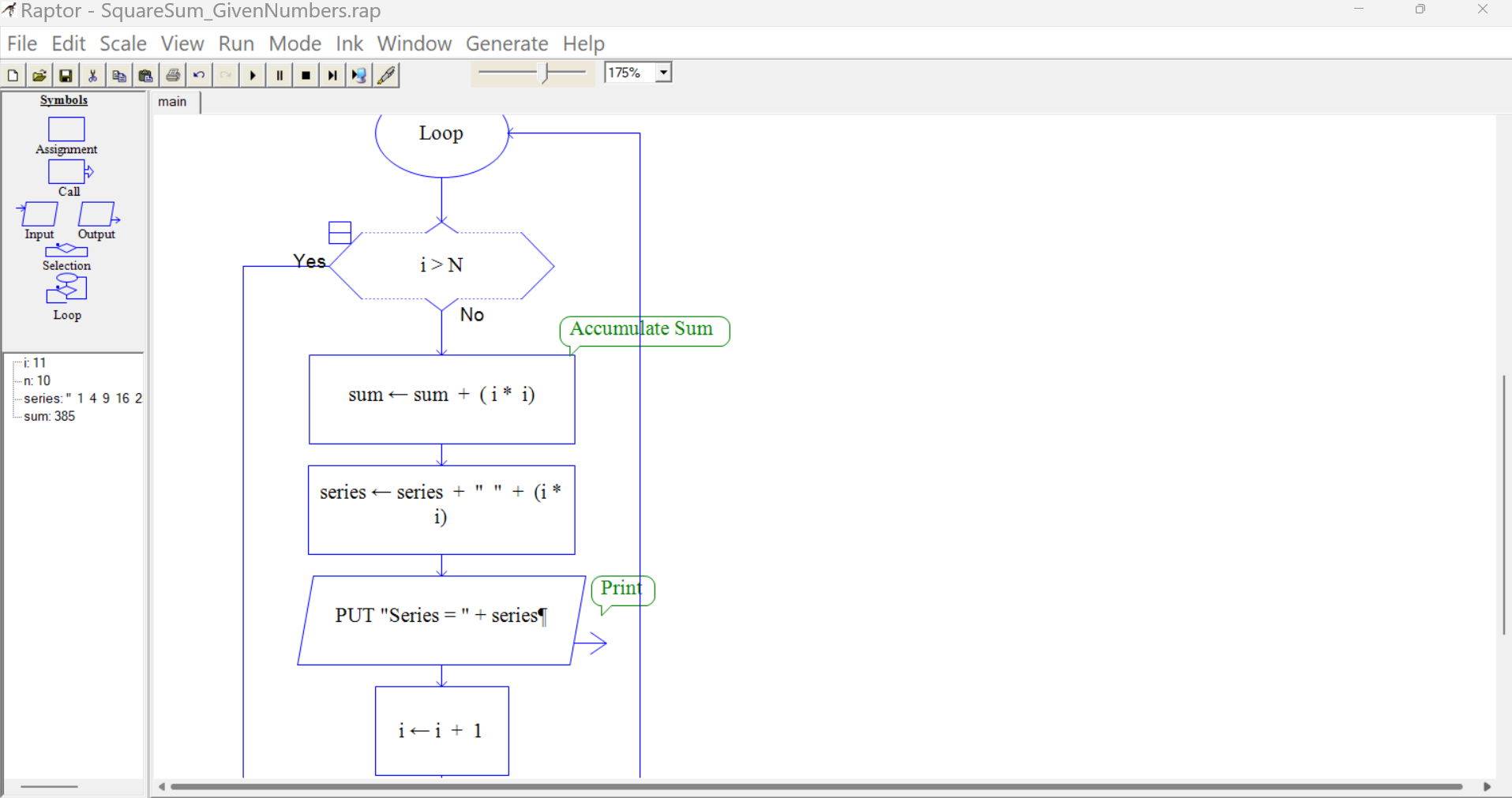## Sum of squares

In the loop structure for the YES decision,
Add an Output symbol to the flowchart to output the sum

Once you are done with a flow chart, we can execute the Raptor flow chart by clicking on Execute to completion button.

We can verify the input and output of the flowchart. ( Explore Run menu options for more details).

## RAPTOR Flowchart

RAPTOR Flowchart for the sum of squares for N integers is as follows: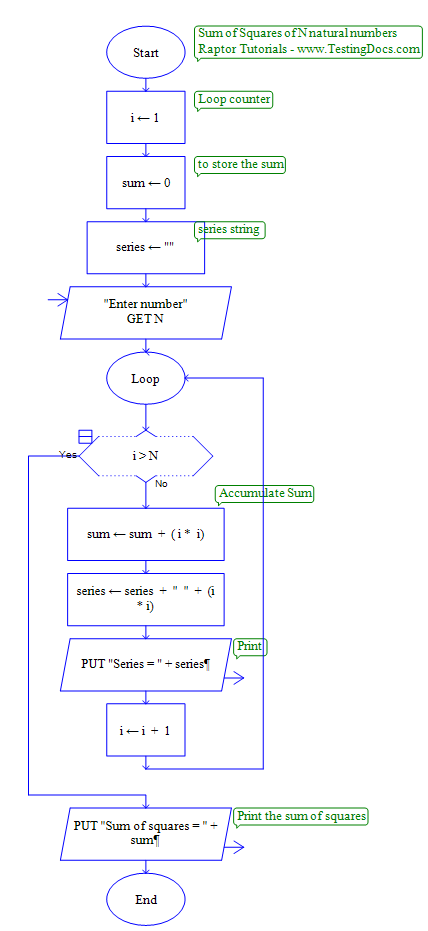## Flowchart Verification

The following Test case is designed to verify the flowchart output.

 Test Case Scenario Detailed Steps Test Data Expected Result Actual Result Find the sum of squares of N integers. Launch Raptor. Model the flowchart. Execute the flowchart. Enter N. 10 385 To be filled after executing the flowchart. The actual result should match the expected result.

## Sample Output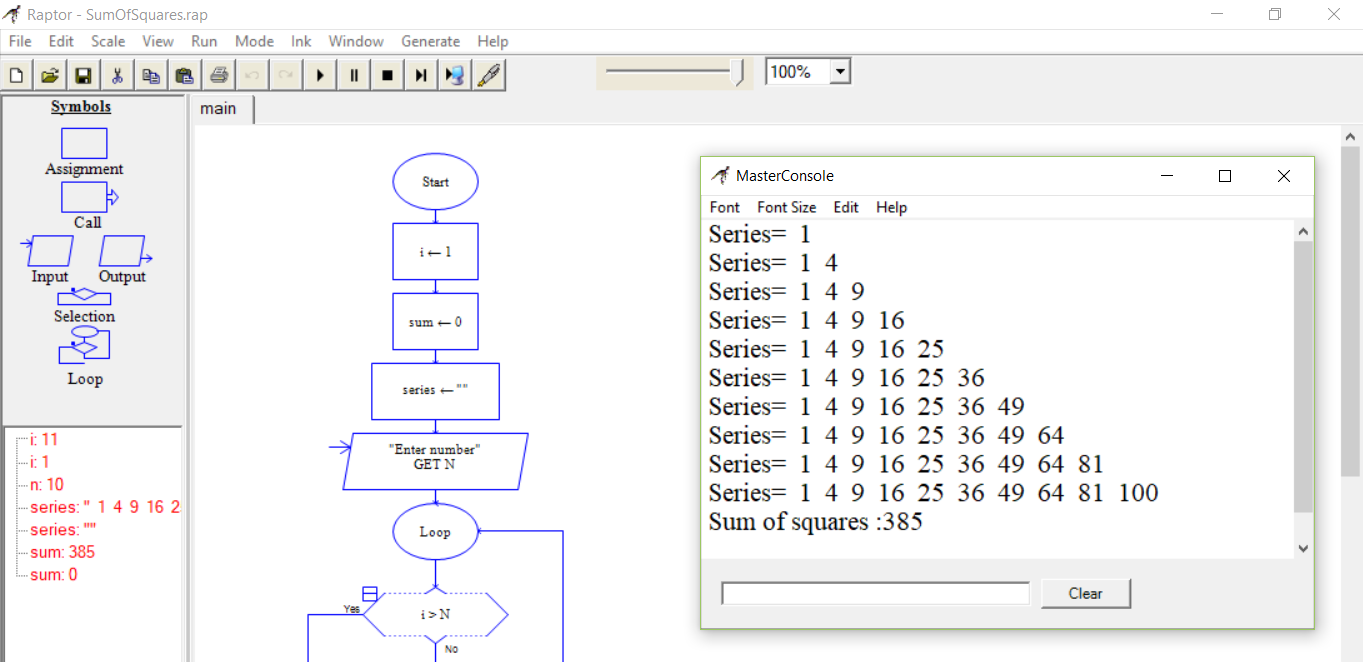Series= 1
Series= 1 4
Series= 1 4 9
Series= 1 4 9 16
Series= 1 4 9 16 25
Series= 1 4 9 16 25 36
Series= 1 4 9 16 25 36 49
Series= 1 4 9 16 25 36 49 64
Series= 1 4 9 16 25 36 49 64 81
Series= 1 4 9 16 25 36 49 64 81 100

Sum of squares:385

That’s it. We have successfully created a flowchart to compute the sum of squares of the first N natural numbers using the RAPTOR flowchart software.

The more advanced flowchart is to compute the squares of the user-given numbers stored in an array. We can design complex flowcharts using procedures and functions to accomplish the sub-tasks.

https://www.testingdocs.com/sum-of-squares-of-given-array-elements/

## Flowchart Examples

https://www.testingdocs.com/raptor-flowchart-examples/

## RAPTOR Tutorial

Raptor Tutorials on this website can be found at:

https://www.testingdocs.com/raptor-a-flowchart-tool/

RAPTOR official website:

https://raptor.martincarlisle.com/